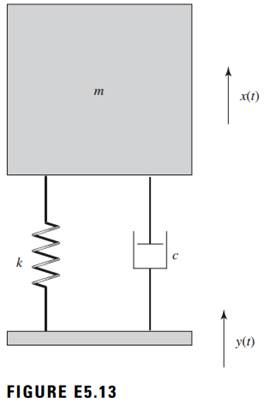### Create an Account

Home / Questions / The damped single degree of freedom mass spring system shown in Figure E513 has a mass m ...

# The damped single degree of freedom mass spring system shown in Figure E513 has a mass m 20 kg and a spring stiffness coefficient k 2400 Nm Determine the damping coefficient of the system

The damped single degree-of-freedom mass spring system shown in Figure E5.13 has a mass m = 20 kg and a spring stiffness coefficient k = 2400 N/m. Determine the damping coefficient of the system, if it is given that the mass exhibits a response with an amplitude of 0.02 m when the support is harmonically excited at the natural frequency of the system with an amplitude Yo = 0.007 m. In addition, determine the amplitude of the dynamic force transmitted to the support.Jun 25 2020 View more View LessSubscribe To Get Solution